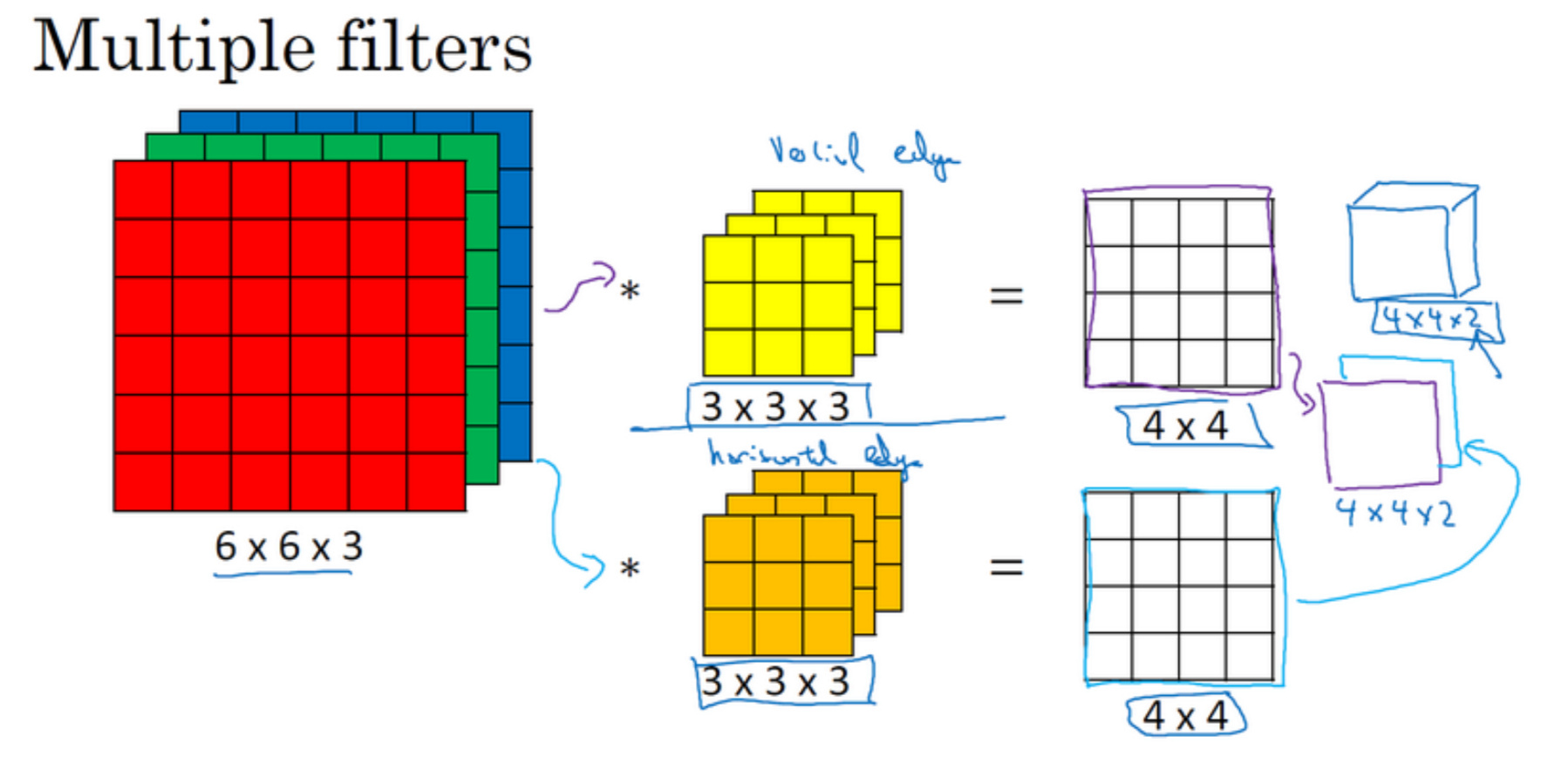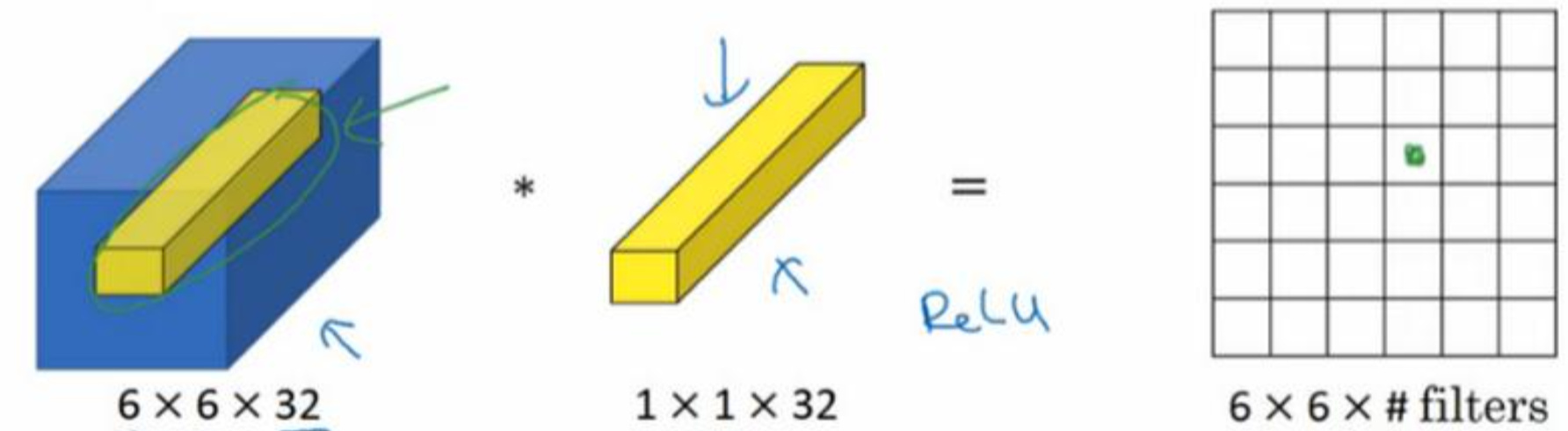## Padding

1. Valid 卷积意味着不填充，这样的话，如果你有一个 $$n × n$$ 的图像，用一个 $$f × f$$ 的过滤器 卷积，它将会给你一个 $$(n − f + 1) × (n − f + 1)$$ 维的输出。
2. 另一个经常被用到的填充方法叫做 Same 卷积，那意味你填充后，你的输出大小和输入 大小是一样的。让 $$𝑛 + 2𝑝 − 𝑓 + 1 = 𝑛$$ 的话，使得输 出和输入大小相等，如果你用这个等式求解 $$p$$ ，那么 $$p =\frac {𝑓 − 1}{2}$$ ，所以当 $$f$$ 是一个奇数的时候，只要选择相应的填充尺寸，你就能确保得到和输入相同尺寸的输出。

## 多维卷积## 1 × 1 卷积

$$1×1$$ 的卷积能做什么呢?不就是乘以数字么?听上去挺好笑的，结果并非如此。